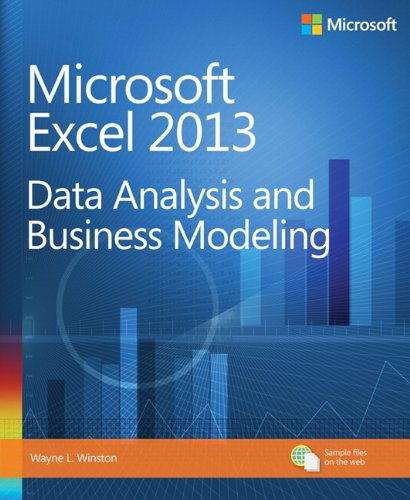# Microsoft Excel 2013 Data Analysis and Business Modeling

Rating: 4.3 out of 5 77 reviews
• Length: 896 pages
• Edition: 1
• Publisher:
• Publication Date: 2014-01-15
• ISBN-10: 0735669139
• ISBN-13: 8601410556181

## Book Description

Master business modeling and analysis techniques with Microsoft Excel 2013, and transform data into bottom-line results. Written by award-winning educator Wayne Winston, this hands-on, scenario-focused guide shows you how to use the latest Excel tools to integrate data from multiple tables—and how to effectively build a relational data source inside an Excel workbook.

• Summarize data with PivotTables and Descriptive Statistics
• Explore new trends in predictive and prescriptive analytics
• Use Excel Trend Curves, multiple regression, and exponential smoothing
• Master advanced Excel functions such as OFFSET and INDIRECT
• Delve into key financial, statistical, and time functions
• Make your charts more effective with the Power View tool
• Tame complex optimization problems with Excel Solver
• Run Monte Carlo simulations on stock prices and bidding models
• Apply important modeling tools such as the Inquire add-in

``````Chapter 1. Range names
Chapter 2. Lookup functions
Chapter 3. INDEX function
Chapter 4. MATCH function
Chapter 5. Text functions
Chapter 6. Dates and date functions
Chapter 7. Evaluating investments by using net present value criteria
Chapter 8. Internal rate of return
Chapter 9. More Excel financial functions
Chapter 10. Circular references
Chapter 11. IF statements
Chapter 12. Time and time functions
Chapter 13. The Paste Special command
Chapter 14. Three-dimensional formulas
Chapter 15. The Auditing tool and Inquire add-in
Chapter 16. Sensitivity analysis with data tables
Chapter 17. The Goal Seek command
Chapter 18. Using the Scenario Manager for sensitivity analysis
Chapter 19. The COUNTIF, COUNTIFS, COUNT, COUNTA, and COUNTBLANK functions
Chapter 20. The SUMIF, AVERAGEIF, SUMIFS, and AVERAGEIFS functions
Chapter 21. The OFFSET function
Chapter 22. The INDIRECT function
Chapter 23. Conditional formatting
Chapter 24. Sorting in Excel
Chapter 25. Tables
Chapter 26. Spinner buttons, scroll bars, option buttons, check boxes, combo boxes, and group list boxes
Chapter 27. The analytics revolution
Chapter 28. Introducing optimization with Excel Solver
Chapter 29. Using Solver to determine the optimal product mix
Chapter 30. Using Solver to schedule your workforce
Chapter 31. Using Solver to solve transportation or distribution problems
Chapter 32. Using Solver for capital budgeting
Chapter 33. Using Solver for financial planning
Chapter 34. Using Solver to rate sports teams
Chapter 35. Warehouse location and the GRG Multistart and Evolutionary Solver engines
Chapter 36. Penalties and the Evolutionary Solver
Chapter 37. The traveling salesperson problem
Chapter 38. Importing data from a text file or document
Chapter 39. Importing data from the Internet
Chapter 40. Validating data
Chapter 41. Summarizing data by using histograms
Chapter 42. Summarizing data by using descriptive statistics
Chapter 43. Using PivotTables and slicers to describe data
Chapter 44. The Data Model
Chapter 45. PowerPivot
Chapter 46. Power View
Chapter 47. Sparklines
Chapter 48. Summarizing data with database statistical functions
Chapter 49. Filtering data and removing duplicates
Chapter 50. Consolidating data
Chapter 51. Creating subtotals
Chapter 52. Charting tricks
Chapter 53. Estimating straight-line relationships
Chapter 54. Modeling exponential growth
Chapter 55. The power curve
Chapter 56. Using correlations to summarize relationships
Chapter 57. Introduction to multiple regression
Chapter 58. Incorporating qualitative factors into multiple regression
Chapter 59. Modeling nonlinearities and interactions
Chapter 60. Analysis of variance: one-way ANOVA
Chapter 61. Randomized blocks and two-way ANOVA
Chapter 62. Using moving averages to understand time series
Chapter 63. Winters’s method
Chapter 64. Ratio-to-moving-average forecast method
Chapter 65. Forecasting in the presence of special events
Chapter 66. An introduction to random variables
Chapter 67. The binomial, hypergeometric, and negative binomial random variables
Chapter 68. The Poisson and exponential random variable
Chapter 69. The normal random variable
Chapter 70. Weibull and beta distributions: modeling machine life and duration of a project
Chapter 71. Making probability statements from forecasts
Chapter 72. Using the lognormal random variable to model stock prices
Chapter 73. Introduction to Monte Carlo simulation
Chapter 74. Calculating an optimal bid
Chapter 75. Simulating stock prices and asset allocation modeling
Chapter 76. Fun and games: simulating gambling and sporting event probabilities
Chapter 77. Using resampling to analyze data
Chapter 78. Pricing stock options
Chapter 79. Determining customer value
Chapter 80. The economic order quantity inventory model
Chapter 81. Inventory modeling with uncertain demand
Chapter 82. Queuing theory: the mathematics of waiting in line
Chapter 83. Estimating a demand curve
Chapter 84. Pricing products by using tie-ins
Chapter 85. Pricing products by using subjectively determined demand
Chapter 86. Nonlinear pricing
Chapter 87. Array formulas and functions``````